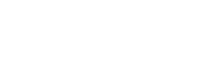Understanding Water Hardness Terminology – Sidon Water### infoeurope@sidonwater.com# Understanding Water Hardness TerminologyThere are several ways you may hear of hard water being described or measured; calcium hardness, magnesium hardness, total hardness, hardness as CaCO3 or total dissolved solids (TDS).  Here is a brief guide to understanding the differences in terminology.

### TDS vs General Water Hardness

Whilst they are measuring different constituents of the water, both TDS and general hardness are directly proportional to each other; the higher the TDS, the harder the water will be.

TDS is the term used to describe the inorganic salts and small amounts of organic matter present in solution in water that cannot be filtered through a filter paper, such as metals, minerals, salts and ions. These are usually calcium, magnesium, sodium, and potassium cations (positively charged ions) and carbonate, hydrogen carbonate, chloride, sulfate, and nitrate anions (negatively charged ions).  TDS measurement is a better reflection of the total mineral content of water rather than the hardness.  General hardness on the other hand, is specifically the combined calcium (Ca2+) and magnesium (Mg2+) concentration in water. While there can be many other ions dissolved in water in addition to calcium and magnesium, such as those mentioned above, these do not count towards general water hardness

### Calcium and Magnesium Hardness

Calcium hardness (calcium carbonate – CaCO3) and magnesium hardness (magnesium carbonate – MgCO3) are measures of the amount of calcium and magnesium ions in water respectively.  Measurements in mg/l give a good indication of the mineral content (and therefore the potential hardness) of your water but they are only measuring one element of the water.  They are however, essential measurements when calculating the total hardness as CaCO3.

### Hardness as CaCO3

Given that the carbonate ion CO32- is common to calcium hardness, magnesium hardness, and alkalinity (alkalinity is water’s ability to neutralise acid and is mainly provided by the carbonate system) and considering that calcium hardness is the most dominant hardness in water (in most cases), it becomes scientifically reasonable to use calcium carbonate (CaCO3) as a unifying entity for the assessment of hardness and alkalinity in water.

### Calculating Hardness as CaCO3

Hardness as CaCO3 is the concentration of calcium and magnesium ions expressed as equivalents of calcium carbonate. Using the periodic table, the molar mass of CaCO3 (rounded to one decimal place) is [40.1+12+(16×3)] = 100.1.  For Ca it is 40.1 and Mg it is 24.3 g/mol.

Therefore, the ratio of calcium carbonate to calcium is 100.1/40.1 = 2.5 and ratio of calcium carbonate to magnesium is 100.1/24.3=4.1.  So, to calculate the hardness as CaCO3 taking both calcium and magnesium measurements into account, you would multiply the calcium measurement by 2.5 and the magnesium measurement by 4.1 and add them together.  For example, for a water analysis report with a magnesium measurement of 1.7 mg/l and a calcium measurement of 117.8 mg/l, the total hardness calculation would be (1.7×4.1) + (117.8×2.5) = 6.97+294.5 = 301.5 mg/l

If you would like to discuss your hard water issues with one of our team we would be pleased to hear from you, contact us today!

Scroll to Top